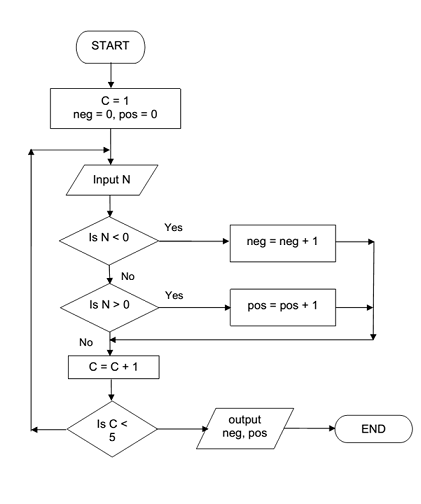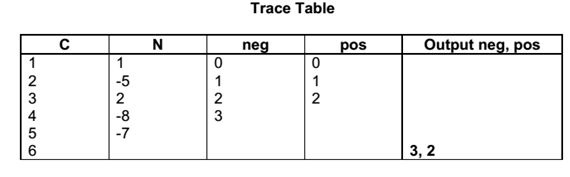## Write the algorithm to find input and output value, Data Structure & Algorithms

Assignment Help:

This algorithm inputs 5 values and outputs how many input numbers were positive and how many were negative.

Data to be used: N = 1, -5, 2, -8, -7#### Binary tree, A binary tree is a tree data structures in which each node hav...

A binary tree is a tree data structures in which each node have at most two child nodes, generally distinguished as "right" and "left". Nodes with children are called parent nodes,

#### Context sensitive f1 help on a field, In what ways we can get the context s...

In what ways we can get the context sensitive F1 help on a field?' Data element documentation. Data element additional text in screen painter. Using the process on help r

#### Advanced data structures, In this unit, the following four advanced data st...

In this unit, the following four advanced data structures have been practically emphasized. These may be considered as alternative to a height balanced tree, i.e., AVL tree.

#### Which sorting algorithms not have running time of o (n2), Which sorting al...

Which sorting algorithms does not have a worst case running time of  O (n 2 ) ? Merge sort

#### Arithmetic expression, How to convert infix postfix and prefix??

How to convert infix postfix and prefix??

#### Mid square method, Mid Square method with good example

Mid Square method with good example

#### B-tree, Unlike a binary-tree, each node of a B-tree may have a number of ke...

Unlike a binary-tree, each node of a B-tree may have a number of keys and children. The keys are stored or saved in non-decreasing order. Each key has an related child that is the

#### Arrays, This unit discussed about data structure called Arrays. The easiest...

This unit discussed about data structure called Arrays. The easiest form of array is a one-dimensional array which may be described as a finite ordered set of homogeneous elements

#### Write the algorithm to find input and output value, This algorithm inputs 5...

This algorithm inputs 5 values and outputs how many input numbers were positive and how many were negative. Data to be used: N = 1, -5, 2, -8, -7

#### Minimum cost spanning trees, A spanning tree of any graph is only a subgrap...

A spanning tree of any graph is only a subgraph that keeps all the vertices and is a tree (having no cycle). A graph might have many spanning trees. Figure: A Graph